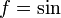# Cotangent function

This article is about a particular function from a subset of the real numbers to the real numbers. Information about the function, including its domain, range, and key data relating to graphing, differentiation, and integration, is presented in the article.
View a complete list of particular functions on this wiki
For functions involving angles (trigonometric functions, inverse trigonometric functions, etc.) we follow the convention that all angles are measured in radians. Thus, for instance, the angle of$90\,^\circ$ is measured as$\pi/2$.

## Definition

The cotangent function, denoted$\cot$, is defined as the pointwise quotient of the cosine function by the sine function:$\! \cot x := \frac{\cos x}{\sin x}$

It can be defined as the composite of the reciprocal function and the tangent function, with the caveat that the cotangent function is defined to be zero at all points where the tangent function is undefined.

## Integration

### First antiderivative: using f'/f formulation

We use the integration form integration of quotient of derivative of function by function:$\int \frac{f'(x)}{f(x)} \, dx = \ln|f(x)| + C$

In our case, we write$\int \cot x \, dx = \int \frac{\cos x}{\sin x} \, dx$

This is the$f'/f$ form where$f = \sin$, so we get:$\! \ln|\sin x| + C$